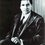# Hardy–Ramanujan number

Srinivasa Ramanujan (22 December 1887 – 26 April 1920) was an Indian mathematician and autodidact.

1729 is the natural number following 1728 and preceding 1730. 1729 is known as the Hardy–Ramanujan number after a famous anecdote of the British mathematician G. H. Hardy regarding a visit to the hospital to see the Indian mathematician Srinivasa Ramanujan. In Hardy's words:

“ I remember once going to see him when he was ill at Putney. I had ridden in taxi cab number 1729 and remarked that the number seemed to me rather a dull one, and that I hoped it was not an unfavorable omen. (instantly)"No," he replied, "it is a very interesting number; it is the smallest number expressible as the sum of two cubes in two different ways." The two different ways are these:

$1729 = 1^{3} + 12^{3} = 9^{3} + 10^{3}$

With almost no formal training in pure mathematics, made extraordinary contributions to mathematical analysis, number theory, infinite series, and continued fractions, Ramanujan developed his own mathematical research in isolation. As a result, he rediscovered known theorems in addition to producing new work. Ramanujan was said to be a natural genius by the English mathematician G. H. Hardy, in the same league as mathematicians such as Euler and Gauss.Note by Vedant Mittal
7 years, 3 months ago

This discussion board is a place to discuss our Daily Challenges and the math and science related to those challenges. Explanations are more than just a solution — they should explain the steps and thinking strategies that you used to obtain the solution. Comments should further the discussion of math and science.

When posting on Brilliant:

• Use the emojis to react to an explanation, whether you're congratulating a job well done , or just really confused .
• Ask specific questions about the challenge or the steps in somebody's explanation. Well-posed questions can add a lot to the discussion, but posting "I don't understand!" doesn't help anyone.
• Try to contribute something new to the discussion, whether it is an extension, generalization or other idea related to the challenge.

MarkdownAppears as
*italics* or _italics_ italics
**bold** or __bold__ bold
- bulleted- list
• bulleted
• list
1. numbered2. list
1. numbered
2. list
Note: you must add a full line of space before and after lists for them to show up correctly
paragraph 1paragraph 2

paragraph 1

paragraph 2

[example link](https://brilliant.org)example link
> This is a quote
This is a quote
    # I indented these lines
# 4 spaces, and now they show
# up as a code block.

print "hello world"
# I indented these lines
# 4 spaces, and now they show
# up as a code block.

print "hello world"
MathAppears as
Remember to wrap math in $$ ... $$ or $ ... $ to ensure proper formatting.
2 \times 3 $2 \times 3$
2^{34} $2^{34}$
a_{i-1} $a_{i-1}$
\frac{2}{3} $\frac{2}{3}$
\sqrt{2} $\sqrt{2}$
\sum_{i=1}^3 $\sum_{i=1}^3$
\sin \theta $\sin \theta$
\boxed{123} $\boxed{123}$

Sort by:

After reading about Ramanujan, I have become a fan of him. He was truly a genius.

- 7 years, 3 months ago

In fact, 1729 is also special because it is the third Carmichael number, which can disprove Fermat's Little Theorem

- 7 years, 3 months ago

yeah you are right

- 7 years, 3 months ago

Carmichael numbers are also great!!

- 6 years, 8 months ago